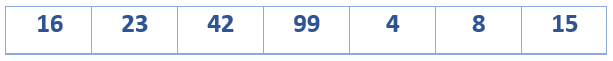Home Arrays Write a program to rotate an array. (Method 2)

# Write a program to rotate an array. (Method 2)

Question: Write a program to rotate an array arr[], having a size ‘n’ by ‘d’ elements?

Input: arr[] = { 4, 8, 15, 16, 23, 42, 99 }, d = 3
Output: 16, 23, 42, 99, 4, 8, 15

Let us suppose our array is in this way:On rotating the array by 3 elements, it should look like:We can do this by rotating the array one element at a time. We discussed it in this post.

We can also use the REVERSAL Algorithm to rotate the array. This can be done as:-

rotate(arr[], d, n)
{
reverse(arr[], 1, d) ;
reverse(arr[], d + 1, n);
reverse(arr[], l, n);
}


Let AB are the two parts of the input array where A = arr[0..d-1] and B = arr[d..n-1]. The idea of the algorithm is:
Reverse A to get ArB. /* Ar is reverse of A */
Reverse B to get ArBr. /* Br is reverse of B */
Reverse all to get (ArBr) r = BA.

For arr[] = [1, 2, 3, 4, 5, 6, 7], d =2 and n = 7
A = [1, 2] and B = [3, 4, 5, 6, 7]
Reverse A, we get ArB = [2, 1, 3, 4, 5, 6, 7]
Reverse B, we get ArBr = [2, 1, 7, 6, 5, 4, 3]
Reverse all, we get (ArBr)r = [3, 4, 5, 6, 7, 1, 2]

Here is the implementation of the same:-

//Function to reverse arr[] from index start to end
void reverseArray(int arr[], int start, int end)
{
int i;
int temp;
while(start < end)
{
temp = arr[start];
arr[start] = arr[end];
arr[end] = temp;
start++;
end--;
}
}

//Function to left rotate arr[] of size n by d
void leftRotate(int arr[], int d, int n)
{
reverseArray(arr, 0, d-1);
reverseArray(arr, d, n-1);
reverseArray(arr, 0, n-1);
}

// Function to print an array
void printArray(int arr[], int size)
{
int i;
for(i = 0; i < size; i++)
printf("%d ", arr[i]);
printf("%\n ");
}

//Driver program to test above functions
int main(void)
{
int arr[] = {4, 8, 15, 16, 23, 42, 99};

leftRotate(arr, 3, 7);
printArray(arr, 7);

return 0;
}


#### You may also likeFebruary 10, 2015 - 09:59

Sorry,its a dumb question …we need to rotate the array itselfFebruary 21, 2015 - 01:16

I hope your query has been resolved :)February 10, 2015 - 09:43

we can also do it by using one auxiliary array and that way the running time will also be minimum.
Is there any problem with that approach other than using extra space ? :P